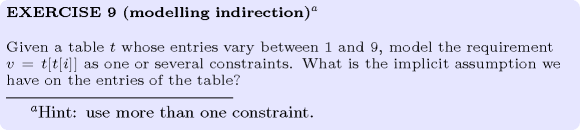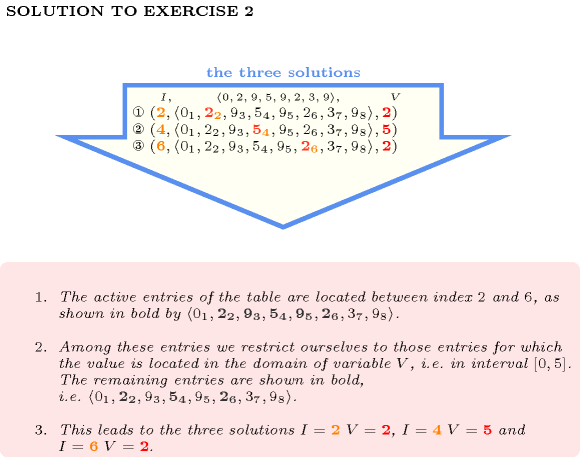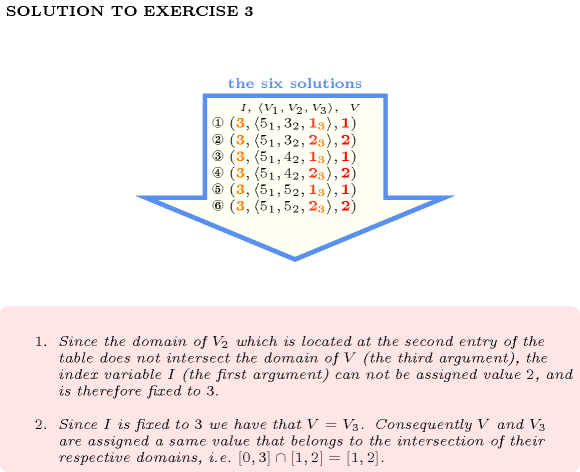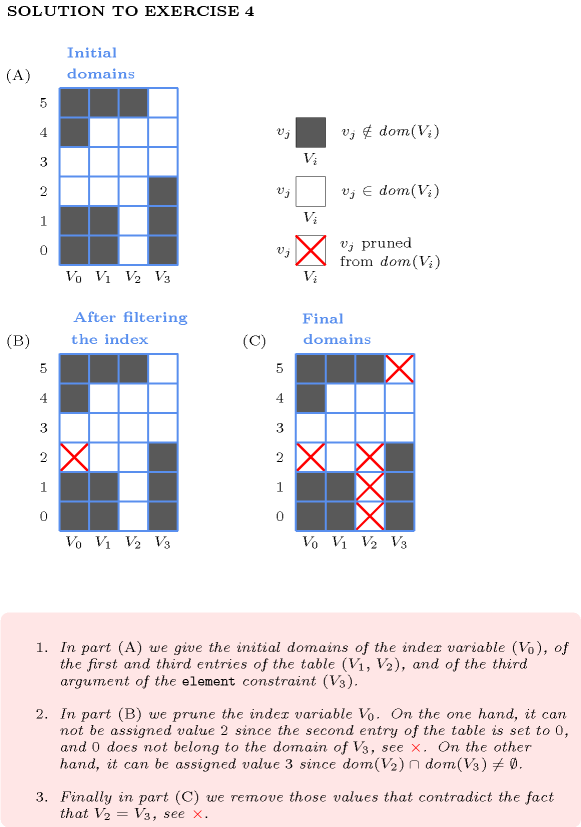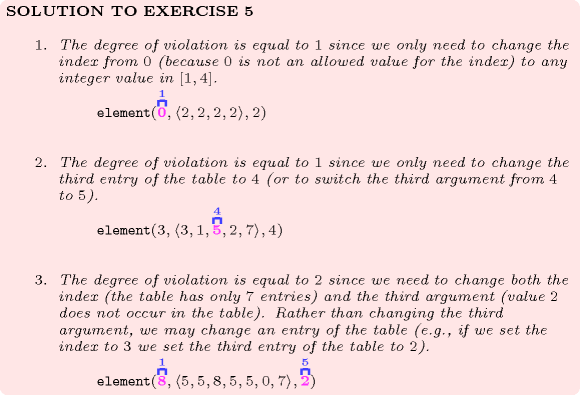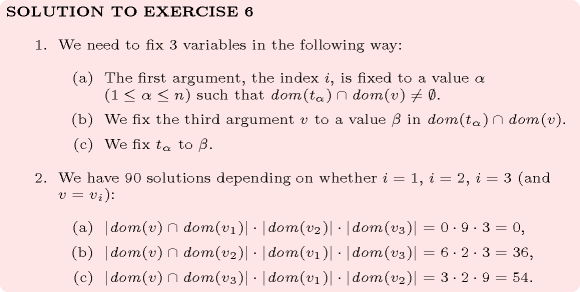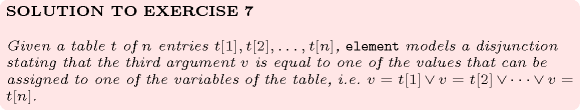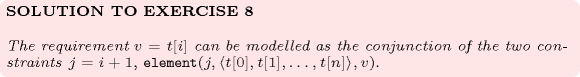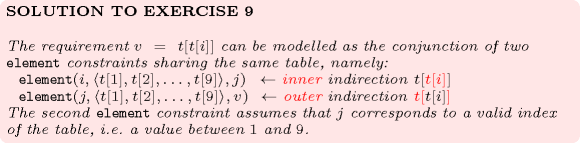## 5.139. element

Origin
Constraint

$\mathrm{𝚎𝚕𝚎𝚖𝚎𝚗𝚝}\left(\mathrm{𝙸𝙽𝙳𝙴𝚇},\mathrm{𝚃𝙰𝙱𝙻𝙴},\mathrm{𝚅𝙰𝙻𝚄𝙴}\right)$

Synonyms

$\mathrm{𝚗𝚝𝚑}$, $\mathrm{𝚎𝚕𝚎𝚖𝚎𝚗𝚝}_\mathrm{𝚟𝚊𝚛}$, $\mathrm{𝚊𝚛𝚛𝚊𝚢}$.

Arguments
 $\mathrm{𝙸𝙽𝙳𝙴𝚇}$ $\mathrm{𝚍𝚟𝚊𝚛}$ $\mathrm{𝚃𝙰𝙱𝙻𝙴}$ $\mathrm{𝚌𝚘𝚕𝚕𝚎𝚌𝚝𝚒𝚘𝚗}\left(\mathrm{𝚟𝚊𝚕𝚞𝚎}-\mathrm{𝚍𝚟𝚊𝚛}\right)$ $\mathrm{𝚅𝙰𝙻𝚄𝙴}$ $\mathrm{𝚍𝚟𝚊𝚛}$
Restrictions
 $\mathrm{𝙸𝙽𝙳𝙴𝚇}\ge 1$ $\mathrm{𝙸𝙽𝙳𝙴𝚇}\le |\mathrm{𝚃𝙰𝙱𝙻𝙴}|$ $|\mathrm{𝚃𝙰𝙱𝙻𝙴}|>0$ $\mathrm{𝚛𝚎𝚚𝚞𝚒𝚛𝚎𝚍}$$\left(\mathrm{𝚃𝙰𝙱𝙻𝙴},\mathrm{𝚟𝚊𝚕𝚞𝚎}\right)$
Purpose

$\mathrm{𝚅𝙰𝙻𝚄𝙴}$ is equal to the ${\mathrm{𝙸𝙽𝙳𝙴𝚇}}^{th}$ item of $\mathrm{𝚃𝙰𝙱𝙻𝙴}$, i.e. $\mathrm{𝚅𝙰𝙻𝚄𝙴}=\mathrm{𝚃𝙰𝙱𝙻𝙴}\left[\mathrm{𝙸𝙽𝙳𝙴𝚇}\right]$.

Example
$\left(3,〈6,9,2,9〉,2\right)$

The $\mathrm{𝚎𝚕𝚎𝚖𝚎𝚗𝚝}$ constraint holds since its third argument $\mathrm{𝚅𝙰𝙻𝚄𝙴}=2$ is equal to the 3th ($\mathrm{𝙸𝙽𝙳𝙴𝚇}=3$) item of the collection $〈6,9,2,9〉$.

All solutions

Figure 5.139.1 gives all solutions to the following non ground instance of the $\mathrm{𝚎𝚕𝚎𝚖𝚎𝚗𝚝}$ constraint: $I\in \left[3,6\right]$, $V\in \left[1,9\right]$, $\mathrm{𝚎𝚕𝚎𝚖𝚎𝚗𝚝}$$\left(I,〈4,8,1,0,3,3,4,3〉,V\right)$.

##### Figure 5.139.1. All solutions corresponding to the non ground example of the $\mathrm{𝚎𝚕𝚎𝚖𝚎𝚗𝚝}$ constraint of the All solutions slot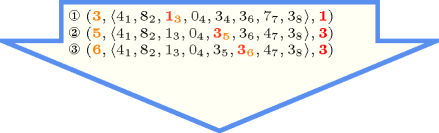Typical
 $|\mathrm{𝚃𝙰𝙱𝙻𝙴}|>1$ $\mathrm{𝚛𝚊𝚗𝚐𝚎}$$\left(\mathrm{𝚃𝙰𝙱𝙻𝙴}.\mathrm{𝚟𝚊𝚕𝚞𝚎}\right)>1$
Symmetry

All occurrences of two distinct values in $\mathrm{𝚃𝙰𝙱𝙻𝙴}.\mathrm{𝚟𝚊𝚕𝚞𝚎}$ or $\mathrm{𝚅𝙰𝙻𝚄𝙴}$ can be swapped; all occurrences of a value in $\mathrm{𝚃𝙰𝙱𝙻𝙴}.\mathrm{𝚟𝚊𝚕𝚞𝚎}$ or $\mathrm{𝚅𝙰𝙻𝚄𝙴}$ can be renamed to any unused value.

Arg. properties
• Functional dependency: $\mathrm{𝚅𝙰𝙻𝚄𝙴}$ determined by $\mathrm{𝙸𝙽𝙳𝙴𝚇}$ and $\mathrm{𝚃𝙰𝙱𝙻𝙴}$.

• Suffix-extensible wrt. $\mathrm{𝚃𝙰𝙱𝙻𝙴}$.

Usage

See Usage slot of $\mathrm{𝚎𝚕𝚎𝚖}$.

Remark

In the original $\mathrm{𝚎𝚕𝚎𝚖𝚎𝚗𝚝}$ constraint of CHIP the $\mathrm{𝚒𝚗𝚍𝚎𝚡}$ attribute was not explicitly present in the table of values. It was implicitly defined as the position of a value in the previous table.

Within some systems (e.g., Gecode), the index of the first entry of the table $\mathrm{𝚃𝙰𝙱𝙻𝙴}$ corresponds to 0 rather than to 1.

When the first entry of the table $\mathrm{𝚃𝙰𝙱𝙻𝙴}$ corresponds to a value $p$ that is different from 1 we can still use the $\mathrm{𝚎𝚕𝚎𝚖𝚎𝚗𝚝}$ constraint. We use the reformulation $I=J-p+1\wedge \mathrm{𝚎𝚕𝚎𝚖𝚎𝚗𝚝}\left(I,\mathrm{𝚃𝙰𝙱𝙻𝙴},V\right)$, where $I$ and $J$ are domain variables respectively ranging from 1 to $|\mathrm{𝚃𝙰𝙱𝙻𝙴}|$ and from $p$ to $p+|\mathrm{𝚃𝙰𝙱𝙻𝙴}|-1$.

The $\mathrm{𝚎𝚕𝚎𝚖𝚎𝚗𝚝}$ constraint is called $\mathrm{𝚗𝚝𝚑}$ in Choco (http://choco.sourceforge.net/). It is also sometimes called $\mathrm{𝚎𝚕𝚎𝚖𝚎𝚗𝚝}_\mathrm{𝚟𝚊𝚛}$ when the second argument corresponds to a table of variables.

The $\mathrm{𝚌𝚊𝚜𝚎}$ constraint [Sicstus95] is a generalisation of the $\mathrm{𝚎𝚕𝚎𝚖𝚎𝚗𝚝}$ constraint, where the table is replaced by a directed acyclic graph describing the set of solutions: there is a one to one correspondence between the solutions and the paths from the unique source of the dag to its leaves.

Systems

generalisation: $\mathrm{𝚌𝚘𝚗𝚍}_\mathrm{𝚕𝚎𝚡}_\mathrm{𝚌𝚘𝚜𝚝}$ ($\mathrm{𝚟𝚊𝚛𝚒𝚊𝚋𝚕𝚎}$ replaced by $\mathrm{𝚝𝚞𝚙𝚕𝚎}$ of $\mathrm{𝚟𝚊𝚛𝚒𝚊𝚋𝚕𝚎𝚜}$).

related: $\mathrm{𝚝𝚠𝚒𝚗}$ ((pairs linked by an element with the same table)).

Keywords
Derived Collection
$\mathrm{𝚌𝚘𝚕}\left(\begin{array}{c}\mathrm{𝙸𝚃𝙴𝙼}-\mathrm{𝚌𝚘𝚕𝚕𝚎𝚌𝚝𝚒𝚘𝚗}\left(\mathrm{𝚒𝚗𝚍𝚎𝚡}-\mathrm{𝚍𝚟𝚊𝚛},\mathrm{𝚟𝚊𝚕𝚞𝚎}-\mathrm{𝚍𝚟𝚊𝚛}\right),\hfill \\ \mathrm{𝚒𝚝𝚎𝚖}\left(\mathrm{𝚒𝚗𝚍𝚎𝚡}-\mathrm{𝙸𝙽𝙳𝙴𝚇},\mathrm{𝚟𝚊𝚕𝚞𝚎}-\mathrm{𝚅𝙰𝙻𝚄𝙴}\right)\right]\hfill \end{array}\right)$
Arc input(s)

$\mathrm{𝙸𝚃𝙴𝙼}$ $\mathrm{𝚃𝙰𝙱𝙻𝙴}$

Arc generator
$\mathrm{𝑃𝑅𝑂𝐷𝑈𝐶𝑇}$$↦\mathrm{𝚌𝚘𝚕𝚕𝚎𝚌𝚝𝚒𝚘𝚗}\left(\mathrm{𝚒𝚝𝚎𝚖},\mathrm{𝚝𝚊𝚋𝚕𝚎}\right)$

Arc arity
Arc constraint(s)
 $•\mathrm{𝚒𝚝𝚎𝚖}.\mathrm{𝚒𝚗𝚍𝚎𝚡}=\mathrm{𝚝𝚊𝚋𝚕𝚎}.\mathrm{𝚔𝚎𝚢}$ $•\mathrm{𝚒𝚝𝚎𝚖}.\mathrm{𝚟𝚊𝚕𝚞𝚎}=\mathrm{𝚝𝚊𝚋𝚕𝚎}.\mathrm{𝚟𝚊𝚕𝚞𝚎}$
Graph property(ies)
$\mathrm{𝐍𝐀𝐑𝐂}$$=1$

Graph model

The original $\mathrm{𝚎𝚕𝚎𝚖𝚎𝚗𝚝}$ constraint with three arguments. We use the derived collection $\mathrm{𝙸𝚃𝙴𝙼}$ for putting together the $\mathrm{𝙸𝙽𝙳𝙴𝚇}$ and $\mathrm{𝚅𝙰𝙻𝚄𝙴}$ parameters of the $\mathrm{𝚎𝚕𝚎𝚖𝚎𝚗𝚝}$ constraint. Within the arc constraint we use the implicit attribute $\mathrm{𝚔𝚎𝚢}$ that associates to each item of a collection its position within the collection.

Parts (A) and (B) of Figure 5.139.2 respectively show the initial and final graph associated with the Example slot. Since we use the $\mathrm{𝐍𝐀𝐑𝐂}$ graph property, the unique arc of the final graph is stressed in bold.

##### Figure 5.139.2. Initial and final graph of the $\mathrm{𝚎𝚕𝚎𝚖𝚎𝚗𝚝}$ constraint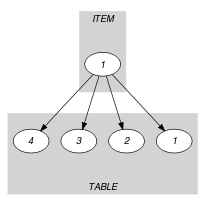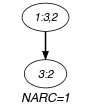(a) (b)
Signature

Because of the first condition of the arc constraint the final graph cannot have more than one arc. Therefore we can rewrite $\mathrm{𝐍𝐀𝐑𝐂}=1$ to $\mathrm{𝐍𝐀𝐑𝐂}\ge 1$ and simplify $\underline{\overline{\mathrm{𝐍𝐀𝐑𝐂}}}$ to $\overline{\mathrm{𝐍𝐀𝐑𝐂}}$.

Automaton

Figure 5.139.3 depicts the automaton associated with the $\mathrm{𝚎𝚕𝚎𝚖𝚎𝚗𝚝}$ constraint. Let ${\mathrm{𝚅𝙰𝙻𝚄𝙴}}_{i}$ be the $\mathrm{𝚟𝚊𝚕𝚞𝚎}$ attribute of item $i$ of the $\mathrm{𝚃𝙰𝙱𝙻𝙴}$ collection. To each triple $\left(\mathrm{𝙸𝙽𝙳𝙴𝚇},\mathrm{𝚅𝙰𝙻𝚄𝙴},{\mathrm{𝚅𝙰𝙻𝚄𝙴}}_{i}\right)$ corresponds a 0-1 signature variable ${S}_{i}$ as well as the following signature constraint: $\left(\mathrm{𝙸𝙽𝙳𝙴𝚇}=i\wedge \mathrm{𝚅𝙰𝙻𝚄𝙴}={\mathrm{𝚅𝙰𝙻𝚄𝙴}}_{i}\right)⇔{S}_{i}$.

##### Figure 5.139.3. Automaton of the $\mathrm{𝚎𝚕𝚎𝚖𝚎𝚗𝚝}$$\left(\mathrm{𝙸𝙽𝙳𝙴𝚇},\mathrm{𝚃𝙰𝙱𝙻𝙴},\mathrm{𝚅𝙰𝙻𝚄𝙴}\right)$ constraint (once one finds the right index and value in the table, one switches from the initial state $s$ to the accepting state $t$)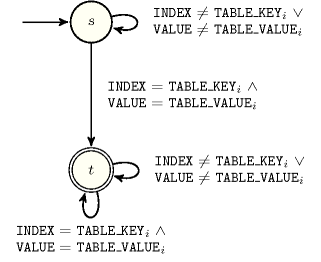##### Figure 5.139.4. Hypergraph of the reformulation corresponding to the automaton of the $\mathrm{𝚎𝚕𝚎𝚖𝚎𝚗𝚝}$ constraint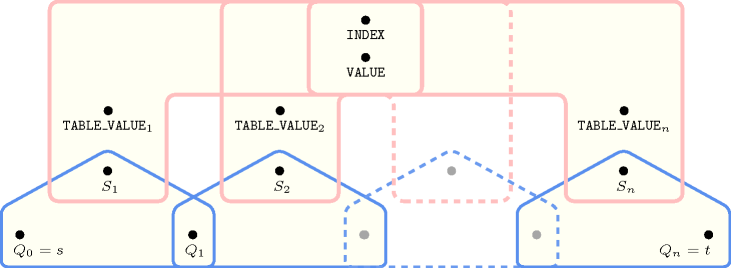Quiz

$\mathrm{𝚎𝚕𝚎𝚖𝚎𝚗𝚝}$: checking whether a ground instance holds or not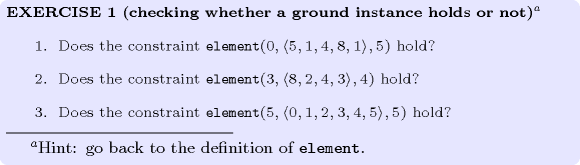$\mathrm{𝚎𝚕𝚎𝚖𝚎𝚗𝚝}$: finding all solutions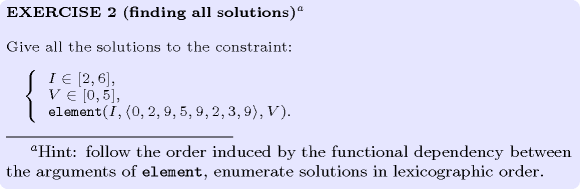$\mathrm{𝚎𝚕𝚎𝚖𝚎𝚗𝚝}$: finding all solutions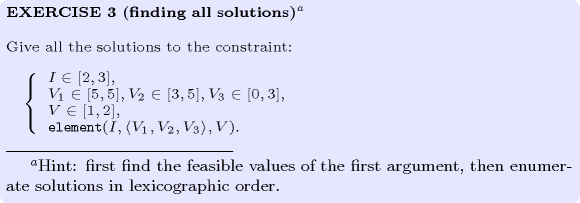$\mathrm{𝚎𝚕𝚎𝚖𝚎𝚗𝚝}$: identifying infeasible values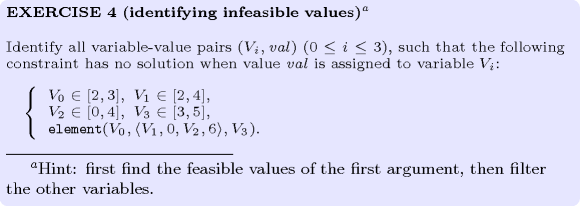$\mathrm{𝚎𝚕𝚎𝚖𝚎𝚗𝚝}$: variable-based degree of violation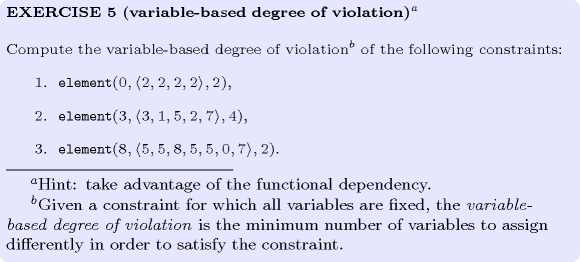$\mathrm{𝚎𝚕𝚎𝚖𝚎𝚗𝚝}$: using entailment for counting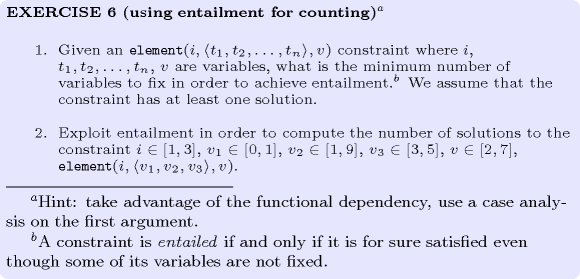$\mathrm{𝚎𝚕𝚎𝚖𝚎𝚗𝚝}$: modelling with an unconstrained index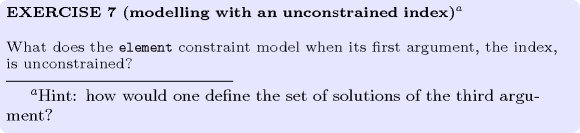$\mathrm{𝚎𝚕𝚎𝚖𝚎𝚗𝚝}$: modelling an index starting at 0$\mathrm{𝚎𝚕𝚎𝚖𝚎𝚗𝚝}$: modelling indirection